Next: Wave Optics Up: Paraxial Optics Previous: Example 13.3: Converging lenses

## Example 13.4: Diverging lenses

Question: How far must an object be placed in front of a diverging lens of focal length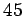cm in order to ensure that the size of the image is fifteen times less than the size of the object? How far in front of the lens is the image located?

Answer: The focal length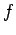of a diverging lens is negative by convention, so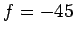cm, in this case. If the image is fifteen times smaller than the object then the magnification is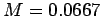. We can be sure that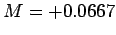, as opposed to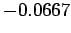, because we know that images formed in diverging lenses are always virtual and upright. According to Eq. (364), the image distanceis given by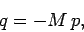where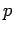is the object distance. This can be combined with Eq. (367) to give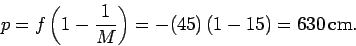Thus, the object must be placed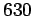cm in front of the lens. The image distance is given by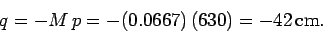Thus, the image is located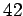cm in front of the lens.Next: Wave Optics Up: Paraxial Optics Previous: Example 13.3: Converging lenses
Richard Fitzpatrick 2007-07-14